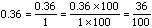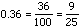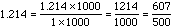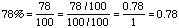Date: Sun, 19 Sep 1999 13:34:27 EDT My son is in 5th grade, and I can't seem to find any papers that have a simple explanation of how to convert decimals into fractions, percents into decimals, and vice-versa. Can you please help? Thank you, Sue Farr Hi Sue, To convert a decimal, like 0.36, to a fraction first think of 0.36 as 0.36 divided by 1. Since a fraction is an integer divided by an integer and the numerator has two digits after the decimal, multiply both the numerator and denominator by 102 = 100. This givesIt is usual to express fractions in lowest terms and both the numerator and denominator are divisible by 4, thusSimilarly,If the decimal is repeating, as in 1.282828..., then there is a Quandaries and Queries note called Repeating Decimals that explains how to convert it to a fraction.    Percent means "per-one-hundred" so converting a decimal to a percent involves converting it to a fraction with 100 in the denominator. Thus, as above, 0.36 = 36/100 or 36% and 1.214 = 121.4/100 or 121.4%.    To convert a percentage to a decimal, again think of the percentage as a fraction with 100 in the denominator. To get a decimal you need to divide the numerator and denominator by 100, thusI hope this helps, Harley

Go to Math Central